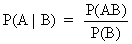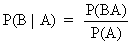Bayes TheoremIntroduction to Econometrics - Bayes Theorem

 As defined earlier(I.II.1) and of course(I.II.2) Since P(A B) = P(B A) it follows by substituting (I.II.2) into (I.II.1) that(I.II.3) Equation (I.II.3) shows clearly that the chance of A given B can be expressed as a function of the chance of B given A! The left hand side of (I.II.3) is called the posterior probability,P(A) is called the prior probability, and P(B) is the probability of B whether A is true or not. It is quite obvious that (I.II.3) can be rewritten as:(I.II.4) where A has been replaced by H (a hypothesis) and B by D (observed data). In other words: the probability of the hypothesis being true, given the fact that specific data have been observed, varies with the likelihood of the observed data when the hypothesis is true times the prior probability (subjective probability) of the hypothesis. As a matter of fact, equation (I.II.4) is a simplified version of Bayes' theorem. By extending (I.II.4) just a little bit, a very useful equation can be found. In fact one only has to think of two different hypotheses Hyp1 and Hyp2 and apply equation (I.II.4) to them. It is easily found that(I.II.5) or in words: the posterior odds equal the likelihood ratio multiplied by the prior odds (Bayes' theorem). The only question remaining is: how can this theorem be applied usefully in practice? A very simple example should clarify the usefulness of this theorem. Suppose that there are two sacks of gold and silver coins. Both have been shuffled thoroughly. Denote the first sack as Hyp1 and the second as Hyp2. Furthermore, it is known that in the first sack there are 150 gold coins and only 50 silver coins, in the other sack there are 100 and 200 coins respectively. Suppose that one had the opportunity to draw a coin out of one sack, and suppose that one would pick a gold coin. What is the chance of the drawn coin to come from the first sack? Assuming that both sacks have the same chance of being picked, not regarded the outcome of the drawing, this problem can be solved quite easily by applying Bayes' theorem as follows:(I.II.6)An important issue, when applying Bayes' theorem or Bayes' regression techniques is that there always is a subjective (prior) probability that is being incorporated in solving the problem. This can be of great importance (e.g., if the researcher has expert knowledge on some events and can therefore influence the outcome of the investigation with his "a priori" assumptions/knowledge). At the same time this prior probability could be regarded as an immense drawback when wrongly used or interpreted. From now on the discussion of classical econometrics will be our primary target. The classical approach does not use a priori knowledge to the full extent as in the Bayes' method, even if there are important economic theories that are known to be true. From now on the economic theory will only be used as a guideline for econometric specifications and checking of econometric models a posteriori.No news at the moment...© 2000-2018 All rights reserved. All Photographs (jpg files) are the property of Corel Corporation, Microsoft and their licensors. We acquired a non-transferable license to use these pictures in this website.
The free use of the scientific content in this website is granted for non commercial use only. In any case, the source (url) should always be clearly displayed. Under no circumstances are you allowed to reproduce, copy or redistribute the design, layout, or any content of this website (for commercial use) including any materials contained herein without the express written permission.

Information provided on this web site is provided "AS IS" without warranty of any kind, either express or implied, including, without limitation, warranties of merchantability, fitness for a particular purpose, and noninfringement. We use reasonable efforts to include accurate and timely information and periodically updates the information without notice. However, we make no warranties or representations as to the accuracy or completeness of such information, and it assumes no liability or responsibility for errors or omissions in the content of this web site. Your use of this web site is AT YOUR OWN RISK. Under no circumstances and under no legal theory shall we be liable to you or any other person for any direct, indirect, special, incidental, exemplary, or consequential damages arising from your access to, or use of, this web site.

Contributions and Scientific Research: Prof. Dr. E. Borghers, Prof. Dr. P. Wessa
Please, cite this website when used in publications: Xycoon (or Authors), Statistics - Econometrics - Forecasting (Title), Office for Research Development and Education (Publisher), http://www.xycoon.com/ (URL), (access or printout date).